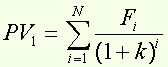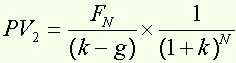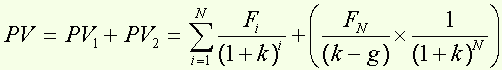DCF valuation

Around the formula...

This method is a way to determine the enterprise value, which is the sum of the present value of after-tax cash flows over the explicit forecast period and the terminal value at the end of the explicit forecast period.

1. Free cash flows calculation

EBIT
- Normalised tax on operating income
+ Depreciation and amortisation
- Capital expenditure
- Change in working capital
= Free cash flow after-tax

The normalised cash flow must be consistent with the assumptions of the business plan :
- The Perpetual growth rate cannot be significantly greater than the long-term growth rate of the economy as a whole,
- The EBITDA margin should be normalised e.g. reflect the return the company will continue to generate to infinity,
- After tax ROCE should not be far from the weighted average cost of capital.

2. Determination of the present value of the free cash flows over the explicit forecast period (from i=1 to i=N)where Fi are the cash flows generated by the security, k is the applied discounting rate (weighted average cost of capital) and N is the number of years for which the security is discounted.

3. Determination of the present value of the terminal value at the end of the explicit forecast period (i=N)where k is the applied discounting rate (wacc) and g the perpetual growth rate, and under the condition that k > g.

4. Enterprise value